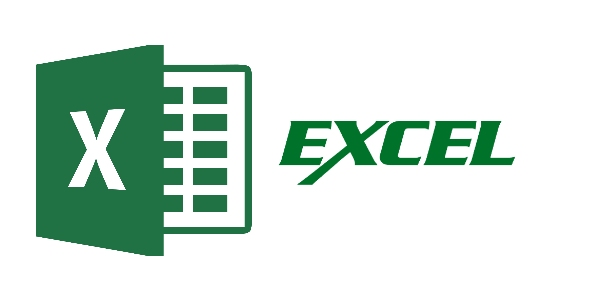# Excel Basic Questions! Trivia Quiz

20 Questions | Total Attempts: 531SettingsBasic Excel Question in this Evaluation. Questions : 20 Time Limit : 40 mins (Max) Pass Criteria : 60 %

• 1.
Which of the following methods cannot be used to edit the content of cell?
• A.

Pressing the Alt key

• B.

Clicking the formula bar

• C.

Pressing F2

• D.

Double clicking the cell

• 2.
Which function calculates your monthly mortage payment?
• A.

PMT (payments)

• B.

NPER (number of periods)

• C.

PV (present value)

• D.

All of above

• 3.
What does the VLOOKUP function do?
• A.

Looks up text that contain ‘v’

• B.

Checks whether text is the same in one cell as in the next

• C.

Finds related records

• D.

All of above

• 4.
Which types of charts can excel produce?
• A.

Line graphs and pie charts only

• B.

Only line graphs

• C.

Bar charts, line graphs and pie charts

• D.

Bar charts and line graphs only

• 5.
Each excel file is called a workbook because
• A.

It can contain text and data

• B.

It can be modified

• C.

It can contain many sheets including worksheets and chart sheets

• D.

You have to work hard to create it

• 6.
Except for the …… function, a formula with a logical function shows the word “TRUE” or “FALSE” as a result
• A.

IF

• B.

AND

• C.

OR

• D.

NOT

• 7.
What function  displays row data in a column or column data in a row
• A.

• B.

Transpose

• C.

Index

• D.

Rows

• 8.
What’s a quick way to extend these numbers to a longer sequence, for instance 1 through 20?
• A.

Select both cells, and then drag the fill handle over the range you want, for instance 18 more rows

• B.

Select the range you want, include both cells, point to fill on the Edit menu, and then click down.

• C.

Copy the second cell, click in the cell below it, on the standard toolbar click the down arrow on the Paste button, and then click Paste Special

• D.

All of above

• 9.
The Name Box
• A.

Shows the location of the previously active cell

• B.

Appears t the left of the formula bar

• C.

Appears below the status bar

• D.

• 10.
• A.

Special shapes like stars and banners

• B.

Drawing objects like rectangles ovals

• C.

Pictures

• D.

None of the Above

• 11.
When you see a cell with a red triangle in the top right corner, what does this signify?
• A.

There is an error in the cell

• B.

There is a comment associated with the cell

• C.

The font color for text in the cell is red

• D.

A formula cannot be entered into the cell

• 12.
What Pivot Table toolbar button updates the data in a Pivot Table or Pivot Chart report if the source data chas changed
• A.

Format Report

• B.

Pivot Table

• C.

Refresh Data

• D.

Show Detail

• 13.
Merge cells option can be applied from
• A.

Format Cells dialog box Alignment Tab

• B.

Home Tab-> alignment->merge

• C.

Ctrl +1

• D.

None of the above

• 14.
When all the numbers between 0 and 100 in a range should be displayed in Red Color, apply
• A.

Use =if() function to format the required numbers red

• B.

Apply Conditional Formatting command on Home Tab in Excel 2010

• C.

Select the cells that contain number between 0 and 100 then click Red color on Text Color tool

• D.

All of above

• 15.
Which function will you use to enter current time in a woksheet cell?
• A.

=today()

• B.

=now()

• C.

=currenttime()

• D.

=time()

• 16.
. To protect a worksheet, you can choose Protection and the Protect Sheet from _______tab
• A.

Edit

• B.

Format

• C.

Review

• D.

Data

• 17.
How are data organized in a spreadsheet?
• A.

Lines and spaces

• B.

Columns and Rows

• C.

Layers and planes

• D.

Height and width

• 18.
Shortcut key to hide entire row
• A.

CTRL +1

• B.

CTRL +6

• C.

CTRL +9

• D.

CTRL +5

• 19.
Which symbol must all formula begin with
• A.

=

• B.

?

• C.

#

• D.

&

• 20.
Intersection of Row and Column is called as
• A.

Cell

• B.

Data

• C.

Field

• D.

Equation

Related TopicsBack to top